# Extension Proof Rules

(Redirected from Extension Rewrite Rules)
Jump to navigationJump to search

Rules that are marked with a * in the first column are implemented in the latest version of Rodin. Rules without a * are planned to be implemented in future versions. Other conventions used in these tables are described in The_Proving_Perspective_(Rodin_User_Manual)#Rewrite_Rules.

### Rewrite Rules

Name Rule Side Condition A/M
*
SIMP_SPECIAL_COND_BTRUE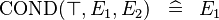$\operatorname{COND}(\btrue, E_1, E_2) \;\;\defi\;\; E_1$ A
*
SIMP_SPECIAL_COND_BFALSE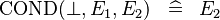$\operatorname{COND}(\bfalse, E_1, E_2) \;\;\defi\;\; E_2$ A
*
SIMP_MULTI_COND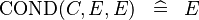$\operatorname{COND}(C, E, E) \;\;\defi\;\; E$ A

### Inference Rules

Name Rule Side Condition A/M
*
DATATYPE_DISTINCT_CASE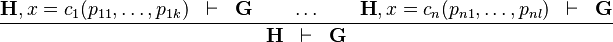$\frac{\textbf{H}, x=c_1(p_{11}, \ldots, p_{1k}) \;\;\vdash \;\; \textbf{G} \qquad \ldots \qquad \textbf{H}, x=c_n(p_{n1}, \ldots, p_{nl}) \;\;\vdash \;\; \textbf{G} }{ \textbf{H} \;\;\vdash\;\; \textbf{G} }$ where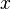$x$ has a datatype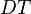$DT$ as type and appears free in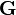$\textbf{G}$,$DT$ has constructors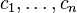$c_1, \ldots, c_n$, parameters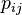$p_{ij}$ are introduced as fresh identifiers M

*
DATATYPE_INDUCTION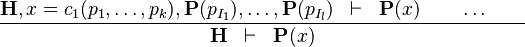$\frac{ \textbf{H}, x=c_1(p_1, \ldots, p_k),\textbf{P}(p_{I_1}), \ldots, \textbf{P}(p_{I_l}) \;\;\vdash \;\; \textbf{P}(x) \qquad \ldots \qquad}{ \textbf{H} \;\;\vdash\;\; \textbf{P}(x) }$ where$x$ has inductive datatype$DT$ as type and appears free in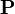$\textbf{P}$;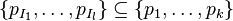$\{p_{I_1}, \ldots, p_{I_l}\} \subseteq \{p_1, \ldots, p_k\}$ are the inductive parameters (if any); an antecedent is created for every constructor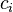$c_i$ of$DT$; all parameters are introduced as fresh identifiers; examples here M